## The Madness of Crowd Models

Our fifth and penultimate post on the 2017 VCE exam madness concerns Question 3 of Section B on the Northern Hemisphere Specialist Mathematics Exam 2. The question begins with the logistic equation for the proportion P of a petri dish covered by bacteria: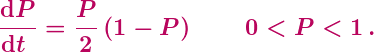This is not a great start, since it’s a little peculiar using the logistic equation to model an area proportion, rather than a population or a population density. It’s also worth noting that the strict inequalities on P are unnecessary and rule out of consideration the equilibrium (constant) solutions P = 0 and P = 1.

Clunky framing aside, part (a) of Question 3 is pretty standard, requiring the solving of the above (separable) differential equation with initial condition P(0) = 1/2. So, a decent integration problem trivialised by the presence of the stupifying CAS machine. After which things go seriously off the rails.

The setting for part (b) of the question has a toxin added to the petri dish at time t = 1, with the bacterial growth then modelled by the equation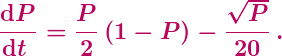Well, probably not. The effect of toxins is most simply modelled as depending linearly on P, and there seems to be no argument for the square root. Still, this kind of fantasy modelling is par for the VCAA‘s crazy course. Then, however, comes Question 3(b):

Find the limiting value of P, which is the maximum possible proportion of the Petri dish that can now be covered by the bacteria.

The question is a mess. And it’s wrong.

The Examiners’ “Report” (which is not a report at all, but merely a list of short answers) fails to indicate what students did or how well they did on this short, 2-mark question. Presumably the intent was for students to find the limit of P by finding the maximal equilibrium solution of the differential equation. So, setting dP/dt = 0 implies that the right hand side of the differential equation is also 0. The resulting equation is not particularly nice, a quartic equation for Q = √P. Just more silly CAS stuff, then, giving the largest solution P = 0.894 to the requested three decimal places.

In principle, applying that approach here is fine. There are, however, two major problems.

The first problem is with the wording of the question: “maximum possible proportion” simply does not mean maximal equilibrium solution, nor much of anything. The maximum possible proportion covered by the bacteria is P = 1. Alternatively, if we follow the examiners and needlessly exclude = 1 from consideration, then there is no maximum possible proportion, and P can just be arbitrarily close to 1. Either way, a large initial P will decay down to the maximal equilibrium solution.

One might argue that the examiners had in mind a continuation of part (a), so that the proportion begins below the equilibrium value and then rises towards it. That wouldn’t rescue the wording, however. The equilibrium solution is still not a maximum, since the equilibrium value is never actually attained. The expression the examiners are missing, and possibly may even have heard of, is least upper bound. That expression is too sophisticated to be used on a school exam, but whose problem is that? It’s the examiners who painted themselves into a corner.

The second issue is that it is not at all obvious – indeed it can easily fail to be true – that the maximal equilibrium solution for P will also be the limiting value of P. The garbled information within question (b) is instructing students to simply assume this. Well, ok, it’s their question. But why go to such lengths to impose a dubious and impossible-to-word assumption, rather than simply asking directly for an equilibrium solution?

To clarify the issues here, and to show why the examiners were pretty much doomed to make a mess of things, consider the following differential equation: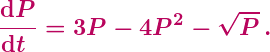By setting Q = √P, for example, it is easy to show that the equilibrium solutions are P = 0 and P = 1/4. Moreover, by considering the sign of dP/dt for P above and below the equilibrium P = 1/4, it is easy to obtain a qualitative sense of the general solutions to the differential equation:In particular, it is easy to see that the constant solution P = 1/4 is a semi-stable equilibrium: if P(0) is slightly below 1/4 then P(t) will decay to the stable equilibrium P = 0.

This type of analysis, which can readily be performed on the toxin equation above, is simple, natural and powerful. And, it seems, non-existent in Specialist Mathematics. The curriculum  contains nothing that suggests or promotes any such analysis, nor even a mention of equilibrium solutions. The same holds for the standard textbook, in which for, for example, the equation for Newton’s law of cooling is solved (clumsily), but there’s not a word of insight into the solutions.

And this explains why the examiners were doomed to fail. Yes, they almost stumbled into writing a good, mathematically rich exam question. The paper thin curriculum, however, wouldn’t permit it.

## A Madness for all Seasons

Our fourth post on the  2017 VCE exam madness will be similar to our previous post: a quick whack of a straight-out error. This error was flagged by a teacher friend, David. (No, not that David.)

The 11th multiple choice question on the first Further Mathematics Exam reads as follows:

Which one of the following statistics can never be negative?

A. the maximum value in a data set

B. the value of a Pearson correlation coefficient

C. the value of a moving mean in a smoothed time series

D. the value of a seasonal index

E. the value of a slope of a least squares line fitted to a scatterplot

Before we get started, a quick word on the question’s repeated use of the redundant “the value of”.

Bleah!

Now, on with answering the question.

It is pretty obvious that the statistics in A, B, C and E can all be negative, so presumably the intended answer is D. However, D is also wrong: a seasonal index can also be negative. Unfortunately the explanation of “seasonal index” in the standard textbook is lost in a jungle of non-explanation, so to illustrate we’ll work through a very simple example.

Suppose a company’s profits and losses over the four quarters of a year are as follows:So, the total profit over the year is $8,000, and then the average quarterly profit is$2000. The seasonal index (SI) for each quarter is then that quarter’s profit (or loss) divided by the average quarterly profit:Clearly this example is general, in the sense that in any scenario where the seasonal data are both positive and negative, some of the seasonal indices will be negative. So, the exam question is not merely technically wrong, with a contrived example raising issues: the question is wrong wrong.

Now, to be fair, this time the VCAA has a defense. It appears to be more common to apply seasonal indices in contexts where all the data are one sign, or to use absolute values to then consider magnitudes of deviations. It also appears that most or all examples Further students would have studied included only positive data.

So, yes, the VCAA (and the Australian Curriculum) don’t bother to clarify the definition or permitted contexts for seasonal indices. And yes, the definition in the standard textbook implicitly permits negative seasonal indices. And yes, by this definition the exam question is plain wrong. But, hopefully most students weren’t paying sufficient attention to realise that the VCAA weren’t paying sufficient attention, and so all is ok.

Well, the defense is something like that. The VCAA can work on the wording.

Our third post on the 2017 VCE exam madness will be brief, on a question containing a flagrant error.

The first question in the matrix module of Further Mathematics’ Exam 2 is concerned with a school canteen selling pies, rolls and sandwiches over three separate weeks. The number of items sold is set up as a 3 x 3 matrix, one row for each week and one column for each food choice. The last part, (c)(ii), of the question then reads:

The matrix equation below shows that the total value of all rolls and sandwiches sold in these three weeks is \$915.60Matrix L in this equation is of order 1 x 3.

Write down matrix L.

This 1-mark question is presumably meant to be a gimme, with answer L = [0 1 1]. Unfortunately the question is both weird and wrong. (And lacking in punctuation. Guys, it’s not that hard.) The wrongness comes from the examiners having confused their rows and columns. As is made clear in the the previous part, (c)(i), of the question, the  3 x 1 matrix of numbers indicates the total earnings from each of the three weeks, not from each of the three food choices. So, the equation indicates the total value of all products sold in weeks 2 and 3.

There’s not much to say about such an obvious error. It is very easy to confuse rows and columns, and we’ve all done it on occasion, but if VCAA’s vetting cannot catch this kind of mistake then it cannot be relied upon to catch anything. The only question is how the Examiners’ Report will eventually address the error. The VCAA is well-practised in cowardly silence and weasel-wording, but it would be exceptionally Trumplike to attempt such tactics here.

Error aside, the question is artificial, and it is not clear that the matrix equation “shows” much of anything. Yes, 0-1 and on-or-off matrices are important and useful, but the use of such a matrix in this context is contrived and confusing. Not a hanging offence, and benign by VCAA’s standards, but the question is pretty silly. And, not forgetting, wrong.

Our second post on the 2017 VCE exam madness concerns a question on the first Specialist Mathematics exam. Typically Specialist exams, particularly the first exams, don’t go too far off the rails; it’s usually more “meh” than madness. (Not that “meh” is an overwhelming endorsement of what is nominally a special mathematics subject.) This year, however, the Specialist exams have some notably Methodsy bits. The following nonsense was pointed out to us by John, a friend and colleague.

The final question, Question 10, on the first Specialist exam concerns the function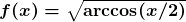, on its maximal domain [-2,2]. In part (c), students are asked to determine the volume of the solid of revolution formed when the region under the graph of f is rotated around the x-axis. This leads to the integralStudents don’t have their stupifying CAS machines in this first exam, so how to do the integral? It is natural to consider integration by parts, but unfortunately this standard and powerful technique is no longer part of the VCE curriculum. (Why not? You’ll have to ask the clowns at ACARA and the VCAA.)

No matter. The VCAA examiners love to have the students to go through a faux-parts computation. So, in part (a) of the question, students are asked to check the derivative of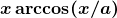. Setting a = 2 in the resulting equation, this givesWe can now integrate and rearrange, giving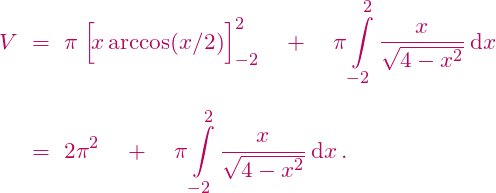So, all that remains is to do that last integral, and … uh oh.

It is easy to integrate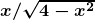indefinitely by substitution, but the problem is that our definite(ish) integral is improper at both endpoints. And, unfortunately, improper integrals are not part of the VCE curriculum. (Why not? You’ll have to ask the clowns at ACARA and the VCAA.) Moreover, even if improper integrals were available, the double improperness is fiddly: we are not permitted to simply integrate from some –b to b and then let b tend to 2.

So, what is a Specialist student to do? One can hope to argue that the integral is zero by odd symmetry, but the improperness is again an issue. As an example indicating the difficulty, the integral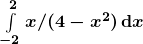is not equal to 0. (The TI Inspire falsely computes the integral to be 0, which is less than inspiring.) Any argument which arrives at the answer 0 for integrating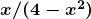is invalid, and is thus prima facie invalid for integratingas well.

Now, in fact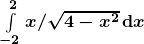is equal to zero, and so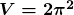. In particular, it is possible to argue that the fatal problem withdoes not occur for our integral, and so both the substitution and symmetry approaches can be made to work. The argument, however, is subtle, well beyond what is expected in a Specialist course.

Note also that this improperness could have been avoided, with no harm to the question, simply by taking the original domain to be, for example, [-1,1]. Which was exactly the approach taken on Question 5 of the 2017 Northern Hemisphere Specialist Exam 1. God knows why it wasn’t done here, but it wasn’t and the consequently the examiners have trouble ahead.

The blunt fact is, Specialist students cannot validly computewith any technique they would have seen in a standard Specialist class. They must either argue incompletely by symmetry or ride roughshod over the improperness. The Examiners’ Report will be a while coming out, though presumably the examiners will accept either argument. But here is a safe prediction: the Report will either contain mealy-mouthed nonsense or blatant mathematical falsehoods. The only alternative is for the examiners to make a clear admission that they stuffed up. Which won’t happen.

Finally, the irony. Look again at the original integral for V. Though this integral arose in the calculation of a volume, it can still be interpreted as the area under the graph of the function y = arccos(x/2):But now we can consider the corresponding area under the inverse function y = 2cos(x):It follows that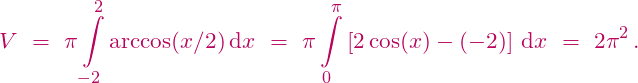Done.

This inverse function trick is standard for Specialist (and Methods) students, and so the students can readily calculate the volume V in this manner. True, reinterpreting the integral for V as an area is a sharp conceptual shift, but with appropriate wording it could have made for a very good Specialist question.

In summary, the Specialist Examiners guided the students to calculate V with a jerry-built technique, leading to an integral that the students cannot validly compute, all the while avoiding a simpler approach well within the students’ grasp. Well played, Examiners, well played.

## There’s Madness in the Methods

Yes, we’ve used that title before, but it’s a damn good title. And there is so much madness in Mathematical Methods to cover. And not only Methods. Victoria’s VCE exams are coming to an end, the maths exams are done, and there is all manner of new and astonishing nonsense to consider. This year, the Victorian Curriculum and Assessment Authority have outdone themselves.

Over the next week we’ll put up a series of posts on significant errors in the 2017 Methods, Specialist Maths and Further Maths exams, including in the mid-year Northern Hemisphere examsBy “significant error” we mean more than just a pointless exercise in button-pushing, or tone-deaf wording, or idiotic pseudomodelling, or aimless pedantry, all of which is endemic in VCE maths exams. A “significant error” in an exam question refers to a fundamental mathematical flaw with the phrasing, or with the intended answer, or with the (presumed or stated) method that students were supposed to use. Not all the errors that we shall discuss are large, but they are all definite errors, they are errors that would have (or at least should have) misled some students, and none of these errors should have occurred. (It is courtesy of diligent (and very annoyed) maths teachers that I learned of most of these questions.) Once we’ve documented the errors, we’ll post on the reasons that the errors are so prevalent, on the pedagogical and administrative climate that permits and encourages them.

Our first post concerns Exam 1 of Mathematical Methods. In the final question, Question 9, students consider the functionon the closed interval [0,1], pictured below. In part (b), students are required to show that, on the open interval (0,1), “the gradient of the tangent to the graph of f” is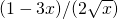. A clumsy combination of calculation and interpretation, but ok. The problem comes when students then have to consider tangents to the graph.In part (c), students take the angle θ in the picture to be 45 degrees. The pictured tangents then have slopes 1 and -1, and the students are required to find the equations of these two tangents. And therein lies the problem: it turns out that the “derivative”  of f is equal to -1 at the endpoint x = 1. However, though the natural domain of the function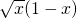is [0,∞), the students are explicitly told that the domain of f is [0,1].

This is obvious and unmitigated madness.

Before we hammer the madness, however, let’s clarify the underlying mathematics.

Does the derivative/tangent of a suitably nice function exist at an endpoint? It depends upon who you ask. If the “derivative” is to exist then the standard “first principles” definition must be modified to be a one-sided limit. So, for our function f above, we would defineThis is clearly not too difficult to do, and with this definition we find that f'(1) = -1, as implied by the Exam question. (Note that since f naturally extends to the right of =1, the actual limit computation can be circumvented.) However, and this is the fundamental point, not everyone does this.

At the university level it is common, though far from universal, to permit differentiability at the endpoints. (The corresponding definition of continuity on a closed interval is essentially universal, at least after first year.) At the school level, however, the waters are much muddier. The VCE curriculum and the most popular and most respected Methods textbook appear to be completely silent on the issue. (This textbook also totally garbles the related issue of derivatives of piecewise defined (“hybrid”) functions.) We suspect that the vast majority of Methods teachers are similarly silent, and that the minority of teachers who do raise the issue would not in general permit differentiability at an endpoint.

In summary, it is perfectly acceptable to permit derivatives/tangents to graphs at their endpoints, and it is perfectly acceptable to proscribe them. It is also perfectly acceptable, at least at the school level, to avoid the issue entirely, as is done in the VCE curriculum, by most teachers and, in particular, in part (b) of the Exam question above.

What is blatantly unacceptable is for the VCAA examiners to spring a completely gratuitous endpoint derivative on students when the issue has never been raised. And what is pure and unadulterated madness is to spring an endpoint derivative after carefully and explicitly avoiding it on the immediately previous part of the question.

The Victorian Curriculum and Assessment Authority has a long tradition of scoring own goals. The question above, however, is spectacular. Here, the VCAA is like a goalkeeper grasping the ball firmly in both hands, taking careful aim, and flinging the ball into his own net.

## Nothing to See Here, Folks###### Image copyright Bodleian Library, University of Oxford

Some pretty cool mathematical history made the news recently. Researchers at Oxford University investigated the Bakhshali manuscript, an ancient Indian text, and using carbon dating they apparently “pin[ned] the moment” of the “discovery of zero”.

Well, no. Dating one particular manuscript to “the 3rd or 4th century [AD]” is not pinpointing anything. And there are other issues.

The story is genuinely interesting, and much of the media reported the conclusions of the (not yet peer-reviewed) research accurately and engagingly. Others, however, muddled the story, particularly in the headlines. In order to clear things up, we can distinguish four related but distinct ideas to which “zero” might refer:

1)(a) The use of some symbol, say , as a placeholder in positional notation. We can then distinguish, for example, 43 and 43 (i.e. four hundred and three).

1)(b) The use of some symbol, say , to represent the number zero, for example in the equation 5 – 5 = .

2)(a) The use of something resembling the symbol 0 as a placeholder (as in 43 versus 403).

2)(b) The use of something resembling the symbol 0 to represent a number (as in 5 – 5 = 0).

All these ideas are of genuine interest, but 1(a) and, particularly, 1(b) much more so. Famously, from about 2000 BC Babylonian mathematicians employed a form of positional notation, using spacing when required to make the positions clear; so, it would be as if we used 43 and 4 3 to indicate forty-three and four hundred and three, respectively. From around 400 BC Babylonian mathematics began to employ a double-wedge symbol as a placeholder. That’s the earliest such occurrence of symbol for “zero”, in any sense, of which we are aware.

It took much longer for zero to be employed as a genuine number. The first known use was in 628 AD, in a text of the Indian mathematician Brahmagupta. He stated algebraic rules of the integers, though in words rather than symbols: a debt [negative] subtracted from zero is a fortune [positive], and so on. The symbolic arithmetic of zero may have followed soon after, though it is not clear (at least to me) even approximately when. By the end of the ninth century, however, the use of the symbol for the number 0 had appeared in both Indian and Arabic arithmetic.

The interest in the Bakhshali Manuscript is its use of (something resembling) the symbol 0: it is the filled-in dot on the bottom line in the photograph above. As for the Babylonians, this dot was employed as a placeholder rather than to represent a number. It had been thought that the Manuscript dated from the ninth century, and more recent than the (placeholder) 0 appearing on the walls of the famous Gwalia Temple, also from the ninth century. The recent carbon dating, however, determined that portions of the Manuscript, including pages that used the dot as a placeholder zero, were much older, dating to around 300 AD. That’s the big news that hit the headlines.

Now, none of that is as mathematically interesting as the still cloudy origins of the number zero. Combined with our knowledge of Brahmagupta, however, this new dating of the Bakhshali Manuscript suggests the possibility that the use of the number 0 in arithmetic occurred centuries earlier than previously suspected. So, not yet the magnificent historical revelation suggested by some newspaper reports, but still very cool.